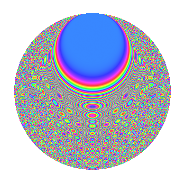# Properties

 Label 2100.2.ciLevel 2100 Weight 2 Character orbit ci Rep. character $$\chi_{2100}(907,\cdot)$$ Character field $$\Q(\zeta_{12})$$ Dimension 576 Sturm bound 960

# Related objects

## Defining parameters

 Level: $$N$$ $$=$$ $$2100 = 2^{2} \cdot 3 \cdot 5^{2} \cdot 7$$ Weight: $$k$$ $$=$$ $$2$$ Character orbit: $$[\chi]$$ $$=$$ 2100.ci (of order $$12$$ and degree $$4$$) Character conductor: $$\operatorname{cond}(\chi)$$ $$=$$ $$140$$ Character field: $$\Q(\zeta_{12})$$ Sturm bound: $$960$$

## Dimensions

The following table gives the dimensions of various subspaces of $$M_{2}(2100, [\chi])$$.

Total New Old
Modular forms 2016 576 1440
Cusp forms 1824 576 1248
Eisenstein series 192 0 192

## Trace form

 $$576q - 24q^{8} + O(q^{10})$$ $$576q - 24q^{8} - 32q^{16} + 64q^{26} + 44q^{28} - 8q^{33} + 12q^{38} + 64q^{41} + 20q^{42} + 24q^{46} + 32q^{48} + 76q^{52} + 64q^{56} + 40q^{58} + 144q^{62} + 48q^{66} + 44q^{68} + 12q^{72} - 16q^{73} + 192q^{76} + 48q^{77} + 48q^{78} + 288q^{81} - 4q^{82} + 8q^{86} - 20q^{88} + 72q^{92} - 16q^{93} - 80q^{97} - 144q^{98} + O(q^{100})$$

## Decomposition of $$S_{2}^{\mathrm{new}}(2100, [\chi])$$ into newform subspaces

The newforms in this space have not yet been added to the LMFDB.

## Decomposition of $$S_{2}^{\mathrm{old}}(2100, [\chi])$$ into lower level spaces

$$S_{2}^{\mathrm{old}}(2100, [\chi]) \cong$$ $$S_{2}^{\mathrm{new}}(140, [\chi])$$$$^{\oplus 4}$$$$\oplus$$$$S_{2}^{\mathrm{new}}(420, [\chi])$$$$^{\oplus 2}$$$$\oplus$$$$S_{2}^{\mathrm{new}}(700, [\chi])$$$$^{\oplus 2}$$

## Hecke characteristic polynomials

There are no characteristic polynomials of Hecke operators in the database